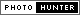## Saturday, April 11, 2009

### PhotoHunt #15 - Trianglethe word triangle means tri - three, and angles. therefore it's all about a closed shape with three angles. Triangles can be classified according to its sides [scalene, isoscles, and equilateral] and angles [acute, obtuse, right, and equiangular].

A scalene triangle has three sides of unequal lengths, an isosceles has two legs/sides of equal lengths, and an equilateral has three sides of equal lengths. That’s for classifying triangles according to its sides. Triangles can also be classified according to its angles: acute triangles has angles that measure less than 90 degrees, an obtuse triangle has an angle measure that’s greater than 90 degrees, a right triangle has an angle measure that is exactly 90 degrees, and lastly, an equilateral triangle has three angles that are equal (60 degrees each measure). The total angle measure of a triangle is 180 degrees!

Triangle is one of the most rigid, fundamental shapes. The pyramids that stand the test of time, has triangular faces... I can list more examples of the use of triangles but I am afraid I’ll bore you. Anyhow, this week's theme on photohunter is Triangle. I am posting a picture of the building with a tower in triangular sections. I go through this route every morning to the babysitter’s house. Tfr!